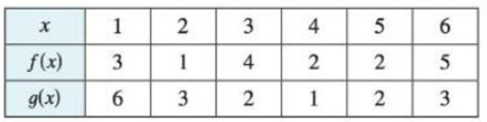Chapter 1.3, Problem 52E### Single Variable Calculus: Early Tr...

8th Edition
James Stewart
ISBN: 9781305270343

#### Solutions

Chapter
Section### Single Variable Calculus: Early Tr...

8th Edition
James Stewart
ISBN: 9781305270343
Textbook Problem

# Use the table to evaluate each expression.(a) f(g(1))(b) g(f(1))(c) f(f(1))(d) g(g(1))(e) (g ◦ f)(3)(f) (f ◦ g)(6)(a)

To determine

To evaluate: The value of f(g(1)) from the given table.

Explanation

From the given table the value of g(1) is 6.

Thus, f(g(1))=f(6)

(b)

To determine

To evaluate: The value of g(f(1)) from the given table.

(c)

To determine

To evaluate: The value of f(f(1)) from the given table.

(d)

To determine

To evaluate: The value of g(g(1)) from the given table.

(e)

To determine

To evaluate: The value of (gf)(3) from the given table.

(f)

To determine

To evaluate: The value of (fg)(6) from the given table.

### Still sussing out bartleby?

Check out a sample textbook solution.

See a sample solution

#### The Solution to Your Study Problems

Bartleby provides explanations to thousands of textbook problems written by our experts, many with advanced degrees!

Get Started

#### In Exercises 516, evaluate the given quantity. log5125

Finite Mathematics and Applied Calculus (MindTap Course List)

#### Place the following set of n = 20 scores in a frequency distribution table.

Essentials of Statistics for The Behavioral Sciences (MindTap Course List)

#### Convert to whole or mixed numbers. 4.

Contemporary Mathematics for Business & Consumers

#### True or False: The slope of a tangent line may be interpreted as average velocity.

Study Guide for Stewart's Single Variable Calculus: Early Transcendentals, 8th